# Solved papers for NEET NEET SOLVED PAPER 2018

### done NEET SOLVED PAPER 2018 Total Questions - 180

• question_answer1) The volume (V) of a monatomic gas varies with its temperature (T), as shown in the graph. The ratio of work done by the gas, to the heat absorbed by it, when it undergoes a change from state A to state B, is [NEET - 2018]A)
$\frac{1}{3}$

B)
$\frac{2}{3}$

C)
$\frac{2}{5}$

D)
$\frac{2}{7}$

• question_answer2) The fundamental frequency in an open organ pipe is equal to the third harmonic of a closed organ pipe. If the length of the closed organ pipe is 20 cm, the length of the open organ pipe is  [NEET - 2018]

A)
12.5 cm

B)
8 cm

C)
13.2 cm

D)
16 cm

• question_answer3) At what temperature will the rms speed of oxygen molecules become just sufficient for escaping from the Earth's atmosphere? (Given: Mass of oxygen molecule $\text{(m)=2}\text{.76 }\!\!\times\!\!\text{ 1}{{\text{0}}^{\text{--26}}}\text{kg}$ Boltzmann's constant ${{\text{k}}_{\text{B}}}\text{=1}\text{.38 }\!\!\times\!\!\text{ 1}{{\text{0}}^{\text{--23}}}\text{J}{{\text{K}}^{\text{--1}}}\text{)}$[NEET - 2018]

A)
$\text{5}\text{.016 }\!\!\times\!\!\text{ 1}{{\text{0}}^{\text{4}}}\text{ K}$

B)
$\text{8}\text{.360 }\!\!\times\!\!\text{ 1}{{\text{0}}^{\text{4}}}\text{ K}$

C)
$\text{2}\text{.508 }\!\!\times\!\!\text{ 1}{{\text{0}}^{\text{4}}}\text{ K}$

D)
$\text{1}\text{.254 }\!\!\times\!\!\text{ 1}{{\text{0}}^{\text{4}}}\text{ K}$

• question_answer4) The efficiency of an ideal heat engine working between the freezing point and boiling point of water, is [NEET - 2018]

A)
6.25%

B)
20%

C)
26.8%

D)
12.5%

• question_answer5) A carbon resistor of$(47\pm 4.7)$ $\text{k}\Omega$ is to be marked with rings of different colours for its identification. The colour code sequence will be          [NEET - 2018]

A)
Yellow - Green - Violet - Gold

B)
Yellow - Violet - Orange - Silver

C)
Violet - Yellow - Orange - Silver

D)
Green - Orange - Violet - Gold

• question_answer6) A set of 'n' equal resistors, of value 'R' each, are connected in series to a battery of emf 'E' and internal resistance 'R'. The current drawn is I. Now, the 'n' resistors are connected in parallel to the same battery. Then the current drawn from battery becomes 10 I. The value of 'n' is               [NEET - 2018]

A)
20

B)
11

C)
10

D)
9

• question_answer7) A battery consists of a variable number 'n' of identical cells (having internal resistance 'r' each) which are connected in series. The terminals of the battery are short-circuited and the current I is measured. Which of the graphs shows the correct relationship between I and n? [NEET - 2018]

A)B)C)D)• question_answer8) Unpolarised light is incident from air on a plane surface of a material of refractive index$'\mu '$. At a particular angle of incidence 'i', it is found that the reflected and refracted rays are perpendicular to each other. Which of the following options is correct for this situation? [NEET - 2018]

A)
$i={{\sin }^{-1}}\left( \frac{1}{\mu } \right)$

B)
Reflected light is polarised with its electric vector perpendicular to the plane of incidence

C)
Reflected light is polarised with its electric vector parallel to the plane of incidence

D)
$i={{\tan }^{-1}}\left( \frac{1}{\mu } \right)$

• question_answer9) In Young's double slit experiment the separation d between the slits is 2 mm, the wavelength $\lambda$ of the light used is $\text{5896}\overset{o}{\mathop{\text{A}}}\,$ and distance D between the screen and slits is 100 cm. It is found that the angular width of the fringes is$\text{0}\text{.20 }\!\!{}^\circ\!\!\text{ }$. To increase the fringe angular width to $\text{0}\text{.21 }\!\!{}^\circ\!\!\text{ }$ (with same $\lambda$ and D) the separation between the slits needs to be changed to [NEET - 2018]

A)
2.1 mm

B)
1.9 mm

C)
1.8 mm

D)
1.7 mm

• question_answer10) An astronomical refracting telescope will have large angular magnification and high angular resolution, when it has an objective lens of [NEET - 2018]

A)
Large focal length and large diameter

B)
Large focal length and small diameter

C)
Small focal length and large diameter

D)
Small focal length and small diameter

• question_answer11) The ratio of kinetic energy to the total energy of an electron in a Bohr orbit of the hydrogen atom, is [NEET - 2018]

A)
2 : -1

B)
1 : -1

C)
1 : 1

D)
1 : -2

• question_answer12) An electron of mass m with an initial velocity$\overrightarrow{v}={{v}_{0}}\widehat{i}({{v}_{0}}>0)$ enters an electric field$\overrightarrow{\text{E}}\text{=-}{{\text{E}}_{\text{0}}}\widehat{\text{i}}\text{(}{{\text{E}}_{\text{0}}}\text{=}$constant > 0) at t = 0. If ${{\lambda }_{0}}$ is its de-Broglie wavelength initially, then its de-Broglie wavelength at time t is            [NEET - 2018]

A)
${{\lambda }_{\text{0}}}\text{t}$

B)
${{\lambda }_{0}}\left( 1+\frac{e{{E}_{0}}}{m{{V}_{0}}}t \right)$

C)
$\frac{{{\lambda }_{0}}}{\left( 1+\frac{e{{E}_{0}}}{m{{V}_{0}}}t \right)}$

D)
${{\lambda }_{0}}$

• question_answer13) For a radioactive material, half-life is 10 minutes. If initially there are 600 number of nuclei, the time taken (in minutes) for the disintegration of 450 nuclei is                         [NEET - 2018]

A)
30

B)
10

C)
20

D)
15

• question_answer14) When the light of frequency $2{{v}_{0}}$(where ${{v}_{0}}$ is threshold frequency), is incident on a metal plate, the maximum velocity of electrons emitted is ${{v}_{1}}$. When the frequency of the incident radiation is increased to$5{{v}_{0}}$, the maximum velocity of electrons emitted from the same plate is ${{v}_{2}}$. The ratio of ${{v}_{1}}$ to ${{v}_{2}}$ is [NEET - 2018]

A)
$4:1$

B)
$1:4$

C)
$1:2$

D)
$2:1$

• question_answer15) In the circuit shown in the figure, the input voltage ${{\text{V}}_{\text{i}}}$ is 20 V, ${{V}_{BE}}=0$and${{V}_{CE}}=0$. The values of ${{\text{I}}_{\text{B}}}\text{,}{{\text{I}}_{\text{C}}}$ and $\beta$ are given by [NEET - 2018]A)
${{I}_{B}}=20\mu A,{{I}_{C}}=5mA,\beta =250$

B)
${{I}_{B}}=25\mu A,{{I}_{C}}=5mA,\beta =200$

C)
${{I}_{B}}=40\mu A,\,\,{{I}_{C}}=10mA,\,\,\beta =250$

D)
${{I}_{B}}=40\mu A,\,\,{{I}_{C}}=5mA,\,\,\beta =125$

• question_answer16) In a p-n junction diode, change in temperature due to heating                        [NEET - 2018]

A)
Does not affect resistance of p-n junction

B)
Affects only forward resistance

C)
Affects only reverse resistance

D)
Affects the overall $\text{V - l}$characteristics of p-n junction

• question_answer17) In the combination of the following gates the output Y can be written in terms of inputs A and B as [NEET - 2018]A)
$\overline{\text{A}\cdot \text{B}}+\text{A}\cdot \text{B}$

B)
$\text{A}\cdot \overline{\text{B}}+\overline{\text{A}}\cdot \text{B}$

C)
$\overline{\text{A}\cdot \text{B}}$

D)
$\overline{\text{A+B}}$

• question_answer18) An em wave is propagating in a medium with a velocity$\overrightarrow{\text{v}}=\text{v}\widehat{\text{i}}$. The instantaneous oscillating electric field of this em wave is along $\text{+y}$ axis. Then the direction of oscillating magnetic field of the em wave will be along [NEET - 2018]

A)
-y direction

B)
+z direction

C)
-z direction

D)
-x direction

• question_answer19) The refractive index of the material of a prism is $\sqrt{2}$ and the angle of the prism is$\text{30 }\!\!{}^\circ\!\!\text{ }$. One of the two refracting surfaces of the prism is made a mirror inwards, by silver coating. A beam of monochromatic light entering the prism from the other face will retrace its path (after reflection from the silvered surface) if its angle of incidence on the prism is                      [NEET - 2018]

A)
$\text{30 }\!\!{}^\circ\!\!\text{ }$

B)
$45{}^\circ$

C)
$60{}^\circ$

D)
Zero

• question_answer20) An object is placed at a distance of 40 cm from a concave mirror of focal length 15 cm. If the object is displaced through a distance of 20 cm towards the mirror, the displacement of the image will be [NEET - 2018]

A)
30 cm towards the mirror

B)
36 cm away from the mirror

C)
30 cm away from the mirror

D)
36 cm towards the mirror

• question_answer21) The magnetic potential energy stored in a certain inductor is 25 mJ, when the current in the inductor is 60 mA. This inductor is of inductance [NEET - 2018]

A)
1.389 H

B)
138.88 H

C)
0.138 H

D)
13.89 H

• question_answer22) An electron falls from rest through a vertical distance h in a uniform and vertically upward directed electric field E. The direction of electric field is now reversed, keeping its magnitude the same. A proton is allowed to fall from rest in it through the same vertical distance h. The time of fall of the electron, in comparison to the time of fall of the proton is  [NEET - 2018]

A)
10 times greater

B)
5 times greater

C)
Smaller

D)
Equal

• question_answer23) The electrostatic force between the metal plates of an isolated parallel plate capacitor C having a charge Q and area A, is [NEET - 2018]

A)
Proportional to the square root of the distance between the plates

B)
Linearly proportional to the distance between the plates

C)
Independent of the distance between the plates

D)
Inversely proportional to the distance between the plates

• question_answer24) A tuning fork is used to produce resonance in a glass tube. The length of the air column in this tube can be adjusted by a variable piston. At room temperature of $\text{27 }\!\!{}^\text{o}\!\!\text{ C}$ two successive resonances are produced at 20 cm and 73 cm of column length. If the frequency of the tuning fork is 320 Hz, the velocity of sound in air at $\text{27 }\!\!{}^\text{o}\!\!\text{ C}$ is      [NEET - 2018]

A)
350 m/s

B)
339 m/s

C)
330 m/s

D)
300 m/s

• question_answer25) A pendulum is hung from the roof of a sufficiently high building and is moving freely to and fro like a simple harmonic oscillator. The acceleration of the bob of the pendulum is $20\text{ }m/{{s}^{2}}$at a distance of 5 m from the mean position. The time period of oscillation is [NEET - 2018]

A)
$\text{2s}$

B)
$\pi \text{s}$

C)
$2\pi \text{s}$

D)
$1\text{s}$

• question_answer26) A metallic rod of mass per unit length $0.5\text{ }kg\text{ }{{m}^{1}}$ is lying horizontally on a smooth inclined plane which makes an angle of $30{}^\circ$ with the horizontal. The rod is not allowed to slide down by flowing a current through it when a magnetic field of induction 0.25 T is acting on it in the vertical direction. The current flowing in the rod to keep it stationary is [NEET - 2018]

A)
$\text{14}\text{.76 A}$

B)
$\text{5}\text{.98 A}$

C)
$\text{7}\text{.14 A}$

D)
$\text{11}\text{.32 A}$

• question_answer27) A thin diamagnetic rod is placed vertically between the poles of an electromagnet. When the current in the electromagnet is switched on, then the diamagnetic rod is pushed up, out of the horizontal magnetic field. Hence the rod gains gravitational potential energy. The work required to do this comes from [NEET - 2018]

A)
The lattice structure of the material of the rod

B)
The magnetic field

C)
The current source

D)
The induced electric field due to the changing magnetic field

• question_answer28) An inductor 20 mH, a capacitor $100\text{ }\mu \text{F}$ and a resistor $50\text{ }\Omega$are connected in series across a source of emf, V=10 sin 314 t. The power loss in the circuit is [NEET - 2018]

A)
2.74 W

B)
0.43 W

C)
0.79 W

D)
1.13 W

• question_answer29) Current sensitivity of a moving coil galvanometer is 5 div/mA and its voltage sensitivity (angular deflection per unit voltage applied) is 20 div/V. The resistance of the galvanometer is [NEET - 2018]

A)
$250\,\,\Omega$

B)
$25\,\,\Omega$

C)
$40\,\,\Omega$

D)
$500\,\,\Omega$

• question_answer30) A body initially at rest and sliding along a frictionless track from a height h (as shown in the figure) just completes a vertical circle of diameter$\text{AB=D}$. The height h is equal to [NEET - 2018]A)
$\frac{7}{5}D$

B)
$D$

C)
$\frac{3}{2}D$

D)
$\frac{5}{4}D$

• question_answer31) Three objects, A : (a solid sphere), B : (a thin circular disk) and C : (a circular ring), each have the same mass M and radius R. They all spin with the same angular speed $\omega$ about their own symmetry axes. The amounts of work (W) required to bring them to rest, would satisfy the relation [NEET - 2018]

A)
${{\text{W}}_{\text{B}}}\text{}{{\text{W}}_{\text{A}}}\text{}{{\text{W}}_{\text{C}}}$

B)
${{\text{W}}_{\text{A}}}\text{}{{\text{W}}_{\text{B}}}\text{}{{\text{W}}_{\text{C}}}$

C)
${{\text{W}}_{\text{C}}}\text{}{{\text{W}}_{\text{B}}}\text{}{{\text{W}}_{\text{A}}}$

D)
${{\text{W}}_{\text{A}}}\text{}{{\text{W}}_{\text{C}}}\text{}{{\text{W}}_{\text{B}}}$

• question_answer32) A moving block having mass m, collides with another stationary block having mass 4m. The lighter block comes to rest after collision. When the initial velocity of the lighter block is v, then the value of coefficient of restitution (e) will be [NEET - 2018]

A)
0.8

B)
0.25

C)
0.5

D)
0.4

• question_answer33) Which one of the following statements is incorrect?     [NEET - 2018]

A)
Frictional force opposes the relative motion.

B)
Limiting value of static friction is directly proportional to normal reaction.

C)
Rolling friction is smaller than sliding friction.

D)
Coefficient of sliding friction has dimensions of length.

• question_answer34) A toy car with charge q moves on a frictionless horizontal plane surface under the influence of a uniform electric field$\overrightarrow{\text{E}}$. Due to the force q$\overrightarrow{\text{E}}$, its velocity increases from 0 to 6 m/s in one second duration. At that instant the direction of the field is reversed. The car continues to move for two more seconds under the influence of this field. The average velocity and the average speed of the toy car between 0 to 3 seconds are respectively [NEET - 2018]

A)
1 m/s, 3.5 m/s

B)
1 m/s, 3 m/s

C)
2 m/s, 4 m/s

D)
1.5 m/s, 3 m/s

• question_answer35) A block of mass m is placed on a smooth inclined wedge ABC of inclination $\theta$ as shown in the figure. The wedge is given an acceleration 'a' towards the right. The relation between a and $\theta$ for the block to remain stationary on the wedge is [NEET - 2018]A)
$a=g\,\,\cos \theta$

B)
$a=\frac{g}{\sin \theta }$

C)
$a=\frac{g}{\text{cosec}\theta }$

D)
$a=g\tan \theta$

• question_answer36) The moment of the force, $\overrightarrow{\text{F}}\text{=4}\widehat{\text{i}}\text{+5}\widehat{\text{j}}\text{-6}\widehat{\text{k}}$ at (2, 0, -3), about the point (2, -2, -2), is given by [NEET - 2018]

A)
$\text{-7}\widehat{\text{i}}\text{-8}\widehat{\text{j}}\text{-4}\widehat{\text{k}}$

B)
$\text{-4}\widehat{\text{i}}\text{-}\widehat{\text{j}}\text{-8}\widehat{\text{k}}$

C)
$\text{-8}\widehat{\text{i}}\text{-4}\widehat{\text{j}}\text{-7}\widehat{\text{k}}$

D)
$\text{-7}\widehat{\text{i}}\text{-4}\widehat{\text{j}}\text{-8}\widehat{\text{k}}$

• question_answer37) A student measured the diameter of a small steel ball using a screw gauge of least count 0.001 cm. The main scale reading is 5 mm and zero of circular scale division coincides with 25 divisions above the reference level. If screw gauge has a zero error of -0.004 cm, the correct diameter of the ball is [NEET - 2018]

A)
0.053 cm

B)
0.525 cm

C)
0.521 cm

D)
0.529 cm

• question_answer38) A solid sphere is rotating freely about its symmetry axis in free space. The radius of the sphere is increased keeping its mass same. Which of the following physical quantities would remain constant for the sphere? [NEET - 2018]

A)
Rotational kinetic energy

B)
Moment of inertia

C)
Angular velocity

D)
Angular momentum

• question_answer39) The kinetic energies of a planet in an elliptical orbit about the Sun, at positions A, B and C are${{\text{K}}_{\text{A}}}$, ${{\text{K}}_{\text{B}}}$ and${{\text{K}}_{\text{C}}}$, respectively. AC is the major axis and SB is perpendicular to AC at the position of the Sun S as shown in the figure. Then                         [NEET - 2018]A)
${{\text{K}}_{\text{B}}}\text{}{{\text{K}}_{\text{A}}}\text{}{{\text{K}}_{\text{C}}}$

B)
${{\text{K}}_{\text{A}}}\text{}{{\text{K}}_{\text{B}}}\text{}{{\text{K}}_{\text{C}}}$

C)
${{\text{K}}_{\text{A}}}\text{}{{\text{K}}_{\text{B}}}\text{}{{\text{K}}_{\text{C}}}$

D)
${{\text{K}}_{\text{B}}}\text{}{{\text{K}}_{\text{A}}}\text{}{{\text{K}}_{\text{C}}}$

• question_answer40) If the mass of the Sun were ten times smaller and the universal gravitational constant were ten times larger in magnitude, which of the following is not correct? [NEET - 2018]

A)
Time period of a simple pendulum on the Earth would decrease

B)
Walking on the ground would become more difficult

C)
Raindrops will fall faster

D)
'g' on the Earth will not change

• question_answer41) A solid sphere is in rolling motion. In rolling motion a body possesses translational kinetic energy $\text{(}{{\text{K}}_{\text{t}}}\text{)}$ as well as rotational kinetic energy $\text{(}{{\text{K}}_{\text{r}}}\text{)}$ simultaneously. The ratio${{\text{K}}_{\text{t}}}\text{:(}{{\text{K}}_{\text{t}}}\text{+}{{\text{K}}_{\text{r}}}\text{)}$ for the sphere is [NEET - 2018]

A)
10 : 7

B)
5 : 7

C)
7 : 10

D)
2 : 5

• question_answer42) A small sphere of radius 'r' falls from rest in a viscous liquid. As a result, heat is produced due to viscous force. The rate of production of heat when the sphere attains its terminal velocity, is proportional to [NEET - 2018]

A)
${{\text{r}}^{\text{5}}}$

B)
${{\text{r}}^{\text{2}}}$

C)
${{\text{r}}^{\text{3}}}$

D)
${{\text{r}}^{\text{4}}}$

• question_answer43) The power radiated by a black body is P and it radiates maximum energy at wavelength, ${{\lambda }_{0}}$. If the temperature of the black body is now changed so that it radiates maximum energy at wavelength$\frac{3}{4}{{\lambda }_{0}}$, the power radiated by it becomes$\text{nP}$. The value of n is [NEET - 2018]

A)
$\frac{256}{81}$

B)
$\frac{4}{3}$

C)
$\frac{3}{4}$

D)
$\frac{81}{256}$

• question_answer44) Two wires are made of the same material and have the same volume. The first wire has cross-sectional area A and the second wire has cross-sectional area 3A. If the length of the first wire is increased by $\Delta /$on applying a force F, how much force is needed to stretch the second wire by the same amount? [NEET - 2018]

A)
4 F

B)
6 F

C)
9 F

D)
F

• question_answer45) A sample of 0.1 g of water at $\text{100 }\!\!{}^\circ\!\!\text{ C}$ and normal pressure $\text{(1}\text{.013 }\!\!\times\!\!\text{ 1}{{\text{0}}^{\text{5}}}\text{N}{{\text{m}}^{\text{--2}}}\text{)}$ requires 54 cal of heat energy to convert to steam at$\text{100 }\!\!{}^\circ\!\!\text{ C}$. If the volume of the steam produced is 167.1 cc, the change in internal energy of the sample, is [NEET - 2018]

A)
42.2 J

B)
208.7 J

C)
104.3 J

D)
84.5 J

• question_answer46) The correct order of N-compounds in its decreasing order of oxidation states is [NEET - 2018]

A)
$\text{HN}{{\text{O}}_{\text{3}}}\text{,N}{{\text{H}}_{\text{4}}}\text{Cl,NO,}{{\text{N}}_{\text{2}}}$

B)
$\text{HN}{{\text{O}}_{\text{3}}}\text{,NO,N}{{\text{H}}_{\text{4}}}\text{Cl,}{{\text{N}}_{\text{2}}}$

C)
$\text{HN}{{\text{O}}_{\text{3}}}\text{,NO,}{{\text{N}}_{\text{2}}}\text{,N}{{\text{H}}_{\text{4}}}\text{Cl}$

D)
$\text{N}{{\text{H}}_{\text{4}}}\text{Cl,}{{\text{N}}_{\text{2}}}\text{,NO,HN}{{\text{O}}_{\text{3}}}$

• question_answer47) Which one of the following elements is unable to form$\text{MF}_{\text{6}}^{\text{3-}}\text{ion}$? [NEET - 2018]

A)
B

B)
Al

C)
Ga

D)
In

• question_answer48) Considering Ellingham diagram, which of the following metals can be used to reduce alumina? [NEET - 2018]

A)
Mg

B)
Zn

C)
Fe

D)
Cu

• question_answer49) The correct order of atomic radii in group 13 elements is                    [NEET - 2018]

A)
$\text{BGaAlTlIn}$

B)
$\text{BAlGaInTl}$

C)
$\text{BAlInGaTl}$

D)
$\text{BGaAlInTl}$

• question_answer50) Which of the following statements is not true for halogens?     [NEET - 2018]

A)
All but fluorine show positive oxidation states

B)
All are oxidizing agents

C)
All form monobasic oxyacid

D)
Chlorine has the highest electron-gain enthalpy

• question_answer51) In the structure of $\text{Cl}{{\text{F}}_{\text{3}}}$, the number of lone pair of electrons on central atom $\text{ }\!\!'\!\!\text{ Cl }\!\!'\!\!\text{ }$ is [NEET - 2018]

A)
Four

B)
Two

C)
One

D)
Three

• question_answer52) Identify the major products P, Q and R in the following sequence of reactions: [NEET - 2018]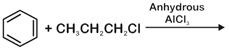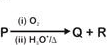A)
 P Q R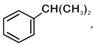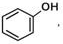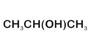B)
 P Q R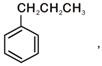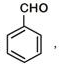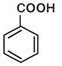C)
 P Q R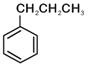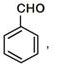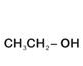D)
 P Q R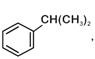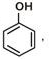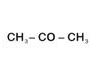• question_answer53) Which of the following compounds can form a zwitterion?      [NEET - 2018]

A)
Benzoic acid

B)
Acetanilide

C)
Aniline

D)
Glycine

• question_answer54) Regarding cross-linked or network polymers, which of the following statements is incorrect? [NEET - 2018]

A)
Examples are bakelite and melamine.

B)
They are formed from bi- and tri-functional monomers.

C)
They contain covalent bonds between various linear polymer chains.

D)
They contain strong covalents bonds in their polymer chains.

• question_answer55) Nitration of aniline in strong acidic medium also gives m-nitroaniline because [NEET - 2018]

A)
In absence of substituents nitro group always goes to m-position.

B)
In electrophilic substitution reactions amino group is meta directive.

C)
Inspite of substituents nitro group always goes to only m-position.

D)
In acidic (strong) medium aniline is present as anilinium ion.

• question_answer56) The difference between amylose and amylopectin is                [NEET - 2018]

A)
Amylopectin have 1$\to$4 $\alpha$-linkage and 1$\to$6 $\beta$-linkage

B)
Amylose have 1$\to$4 $\alpha$-linkage and 1$\to$6 $\beta$-linkage

C)
Amylopectin have 1$\to$4 $\alpha$-linkage and 1$\to$6$\alpha$-linkage

D)
Amylose is made up of glucose and galactose

• question_answer57) A mixture of 2.3 g formic acid and 4.5 g oxalic acid is treated with conc. ${{\text{H}}_{\text{2}}}\text{S}{{\text{o}}_{\text{4}}}$. The evolved gaseous mixture is passed through KOH pellets. Weight (in g) of the remaining product at STP will be     [NEET - 2018]

A)
2.8

B)
3.0

C)
1.4

D)
4.4

• question_answer58) Which of the following oxides is most acidic in nature?           [NEET - 2018]

A)
$\text{BaO}$

B)
$\text{BeO}$

C)
$\text{MgO}$

D)
$\text{CaO}$

• question_answer59) Which oxide of nitrogen is not a common pollutant introduced into the atmosphere both due to natural and human activity? [NEET - 2018]

A)
${{\text{N}}_{\text{2}}}\text{O}$

B)
$\text{N}{{\text{O}}_{2}}$

C)
${{\text{N}}_{\text{2}}}{{\text{O}}_{\text{5}}}$

D)
$\text{NO}$

• question_answer60) The compound A on treatment with Na gives B, and with $\text{PC}{{\text{l}}_{\text{5}}}$ gives C. B and C react together to give diethyl ether. A, B and C are in the order                       [NEET - 2018]

A)
${{\text{C}}_{\text{2}}}{{\text{H}}_{\text{5}}}\text{Cl, }{{\text{C}}_{\text{2}}}{{\text{H}}_{\text{6}}}\text{,}{{\text{C}}_{\text{2}}}{{\text{H}}_{\text{5}}}\text{OH}$

B)
${{\text{C}}_{\text{2}}}{{\text{H}}_{\text{5}}}\text{OH, }{{\text{C}}_{\text{2}}}{{\text{H}}_{\text{5}}}\text{Cl,}{{\text{C}}_{\text{2}}}{{\text{H}}_{\text{5}}}\text{ONa}$

C)
${{\text{C}}_{\text{2}}}{{\text{H}}_{\text{5}}}\text{OH,}{{\text{C}}_{\text{2}}}{{\text{H}}_{\text{6}}}\text{,}{{\text{C}}_{\text{2}}}{{\text{H}}_{\text{5}}}\text{Cl}$

D)
${{\text{C}}_{\text{2}}}{{\text{H}}_{\text{5}}}\text{OH, }{{\text{C}}_{\text{2}}}{{\text{H}}_{\text{5}}}\text{ONa, }{{\text{C}}_{\text{2}}}{{\text{H}}_{\text{5}}}\text{Cl}$

• question_answer61) The compound ${{\text{C}}_{\text{7}}}{{\text{H}}_{\text{8}}}$ undergoes the following reactions$:{{\text{C}}_{\text{7}}}{{\text{H}}_{\text{8}}}\xrightarrow{3C{{l}_{2}}/\Delta }A\xrightarrow{B{{r}_{2}}/Fe}B\xrightarrow{Zn/HCl}C$. The product 'C' is [NEET - 2018]

A)
3-bromo-2, 4, 6-trichlorotoluene

B)
o-bromotoluene

C)
m-bromotoluene

D)
p-bromotoluene

• question_answer62) Hydrocarbon [A] reacts with bromine by substitution to form an alkyl bromide which by Wurtz reaction is converted to gaseous hydrocarbon containing less than four carbon atoms. [A] is          [NEET - 2018]

A)
$\text{C}{{\text{H}}_{\text{3}}}\text{-C}{{\text{H}}_{\text{3}}}$

B)
$\text{C}{{\text{H}}_{\text{2}}}\text{=C}{{\text{H}}_{\text{2}}}$

C)
$\text{CH}\equiv \text{CH}$

D)
$\text{C}{{\text{H}}_{\text{4}}}$

• question_answer63) Which of the following molecules represents the order of hybridisation $\text{s}{{\text{p}}^{\text{2}}}\text{, s}{{\text{p}}^{\text{2}}}\text{, sp, sp}$from left to right atoms?               [NEET - 2018]

A)
$\text{C}{{\text{H}}_{\text{2}}}\text{=CH-CH=C}{{\text{H}}_{\text{2}}}$

B)
$\text{C}{{\text{H}}_{\text{2}}}\text{=CH-C}\equiv \text{CH}$

C)
$\text{HC}\equiv \text{C-C}\equiv \text{CH}$

D)
$\text{C}{{\text{H}}_{\text{3}}}\text{-CH=CH-C}{{\text{H}}_{\text{3}}}$

• question_answer64) Which of the following carbocation?s is expected to be most stable? [NEET - 2018]

A)B)C)D)• question_answer65) Which of the following is correct with respect to - I effect of the substituents? (R = alkyl)                         [NEET - 2018]

A)
$\text{-N}{{\text{H}}_{\text{2}}}\text{-OR-F}$

B)
$\text{-N}{{\text{R}}_{\text{2}}}\text{-OR-F}$

C)
$\text{-N}{{\text{H}}_{\text{2}}}\text{-OR-F}$

D)
$\text{-N}{{\text{R}}_{\text{2}}}\text{-OR-F}$

• question_answer66) In the reaction. The electrophile involved is [NEET - 2018]

A)
Dichloromethyl anion \left( \begin{align} & \oplus \\ & \text{CHC}{{\text{l}}_{\text{2}}} \\ \end{align} \right)

B)
Formyl cation \left( \begin{align} & \oplus \\ & \text{CHO} \\ \end{align} \right)

C)
Dichloromethyl cation \left( \begin{align} & \oplus \\ & \text{CHC}{{\text{l}}_{2}} \\ \end{align} \right)

D)
Dichlorocarbene $\text{(:CC}{{\text{l}}_{\text{2}}})$

• question_answer67) Carboxylic acids have higher boiling points than aldehydes, ketones and even alcohols of comparable molecular mass. It is due to their [NEET - 2018]

A)
More extensive association of carboxylic acid via van der Waals force of attraction

B)
Formation of carboxylate ion

C)
Formation of intramolecular H-bonding

D)
Formation of intermolecular H-bonding

• question_answer68) Compound $\text{A,}{{\text{C}}_{\text{8}}}{{\text{H}}_{\text{10}}}\text{O,}$ is found to react with $\text{NaOI}$ (produced by reacting Y with$\text{NaOH}$) and yields a yellow precipitate with characteristic smell. A and Y are respectively [NEET - 2018]

A)B)C)D)• question_answer69) Match the metal ions given in Column I with the spin magnetic moments of the ions given in Column II and assign the correct code:                         [NEET - 2018]  Column I Column II a. $\text{C}{{\text{o}}^{\text{3+}}}$ i. $\sqrt{\text{8}}\text{ BM}$ b. $\text{C}{{\text{r}}^{\text{3+}}}$ ii. $\sqrt{35}\text{ }BM$ c. $\text{F}{{\text{e}}^{\text{3+}}}$ iii. $\sqrt{3}\text{ }BM$ d. $\text{N}{{\text{i}}^{\text{2+}}}$ iv. $\sqrt{24}\text{ }BM$ v. $\sqrt{15}\text{ }BM$

A)
a-iv      b-i        c-ii       d-iii

B)
a-I        b-ii       c-iii      d-iv

C)
a-iv      b-v       c-ii       d-i

D)
a-iii      b-v       c-I        d-ii

• question_answer70) Which one of the following ions exhibits d-d transition and paramagnetism as well? [NEET - 2018]

A)
$\text{MnO}_{\text{4}}^{\text{-}}$

B)
$\text{C}{{\text{r}}_{\text{2}}}\text{O}_{\text{7}}^{\text{2-}}$

C)
$\text{CrO}_{4}^{\text{2-}}$

D)
$\text{MnO}_{\text{4}}^{\text{2-}}$

• question_answer71) Iron carbonyl, $\text{Fe(CO}{{\text{)}}_{\text{5}}}$ is [NEET - 2018]

A)
Trinuclear

B)
Mononuclear

C)
Tetranuclear

D)
Dinuclear

• question_answer72) The type of isomerism shown by the complex $\text{ }\!\![\!\!\text{ CoC}{{\text{l}}_{\text{2}}}{{\text{(en)}}_{\text{2}}}\text{ }\!\!]\!\!\text{ }$is [NEET - 2018]

A)
Ionization isomerism

B)
Coordination isomerism

C)
Geometrical isomerism

D)

• question_answer73) The geometry and magnetic behaviour of the complex $\text{ }\!\![\!\!\text{ Ni(CO}{{\text{)}}_{\text{4}}}\text{ }\!\!]\!\!\text{ }$ are [NEET - 2018]

A)
Square planar geometry and paramagnetic

B)
Tetrahedral geometry and diamagnetic

C)
Square planar geometry and diamagnetic

D)
Tetrahedral geometry and paramagnetic

• question_answer74) Following solutions were prepared by mixing different volumes of NaOH and HCl of different concentrations :  [NEET - 2018]  A. $\text{60mL}\frac{\text{M}}{\text{10}}\text{HCl+40mL}\frac{\text{M}}{\text{10}}\text{NaOH}$ B. $\text{55mL}\frac{\text{M}}{\text{10}}\text{HCl+45mL}\frac{\text{M}}{\text{10}}\text{NaOH}$ C. $\text{75mL}\frac{\text{M}}{\text{5}}\text{HCl+25mL}\frac{\text{M}}{\text{5}}\text{NaOH}$ D. $\text{100mL}\frac{\text{M}}{\text{10}}\text{HCl+100mL}\frac{\text{M}}{\text{10}}\text{NaOH}$
pH of which one of them will be equal to 1?

A)
D

B)
A

C)
B

D)
C

• question_answer75) On which of the following properties does the coagulating power of an ion depend? [NEET - 2018]

A)
Both magnitude and sign of the charge on the ion

B)
Size of the ion alone

C)
The magnitude of the charge on the ion alone

D)
The sign of charge on the ion alone

• question_answer76) Given van der Waals constant for $\text{N}{{\text{H}}_{\text{3}}}\text{,}{{\text{H}}_{\text{2}}}\text{,}{{\text{O}}_{\text{2}}}$ and $\text{C}{{\text{O}}_{2}}$ are respectively 4.17, 0.244, 1.36 and 3.59, which one of the following gases is most easily liquefied?                       [NEET - 2018]

A)
${{\text{O}}_{\text{2}}}$

B)
${{\text{H}}_{\text{2}}}$

C)
$\text{N}{{\text{H}}_{3}}$

D)
$\text{C}{{\text{O}}_{\text{2}}}$

• question_answer77) The solubility of $\text{BaS}{{\text{O}}_{\text{4}}}$ in water is $\text{2}\text{.42 }\!\!\times\!\!\text{ 1}{{\text{0}}^{\text{--3}}}\text{g}{{\text{L}}^{\text{-1}}}$ at 298 K. The value of its solubility product$\text{(}{{\text{K}}_{\text{sp}}}\text{)}$ will be  (Given molar mass of$\text{BaS}{{\text{O}}_{\text{4}}}\text{=233 g mo}{{\text{l}}^{\text{--1}}}$)[NEET - 2018]

A)
$\text{1}\text{.08 }\!\!\times\!\!\text{ 1}{{\text{0}}^{\text{--14}}}\text{ mol 2}{{\text{L}}^{\text{--2}}}$

B)
$\text{1}\text{.08 }\!\!\times\!\!\text{ 1}{{\text{0}}^{\text{--12}}}\text{mo}{{\text{l}}^{\text{2}}}{{\text{L}}^{\text{--2}}}$

C)
$\text{1}\text{.08 }\!\!\times\!\!\text{ 1}{{\text{0}}^{\text{--10}}}\text{mo}{{\text{l}}^{\text{2}}}{{\text{L}}^{\text{--2}}}$

D)
$\text{1}\text{.08 }\!\!\times\!\!\text{ 1}{{\text{0}}^{\text{--8}}}\text{mo}{{\text{l}}^{\text{2}}}{{\text{L}}^{\text{--2}}}$

• question_answer78) In which case is number of molecules of water maximum?      [NEET - 2018]

A)
0.00224 L of water vapours at 1 atm and 273 K

B)
0.18 g of water

C)
18 mL of water

D)
$\text{1}{{\text{0}}^{\text{--3}}}\text{ mol}$of water

• question_answer79) The correct difference between first and second order reactions is that                       [NEET - 2018]

A)
A first-order reaction can catalyzed; a second-order reaction cannot be catalyzed

B)
The half-life of a first-order reaction does not depend on${{\text{ }\!\![\!\!\text{ A }\!\!]\!\!\text{ }}_{0}}$; the half-life of a second-order reaction does depend on ${{\text{ }\!\![\!\!\text{ A }\!\!]\!\!\text{ }}_{0}}$

C)
The rate of a first-order reaction does not depend on reactant concentrations; the rate of a second-order reaction does depend on reactant concentrations

D)
The rate of a first-order reaction does depend on reactant concentrations; the rate of a second-order reaction does not depend on reactant concentrations.

• question_answer80) Among$\text{Ca}{{\text{H}}_{\text{2}}}$, $\text{Be}{{\text{H}}_{\text{2}}}$, $\text{Ba}{{\text{H}}_{\text{2}}}$, the order of ionic character is [NEET - 2018]

A)
$\text{Be}{{\text{H}}_{\text{2}}}\text{ Ba}{{\text{H}}_{\text{2}}}\text{ Ca}{{\text{H}}_{\text{2}}}$

B)
$\text{Ca}{{\text{H}}_{\text{2}}}\text{ Be}{{\text{H}}_{\text{2}}}\text{ Ba}{{\text{H}}_{\text{2}}}$

C)
$\text{Be}{{\text{H}}_{\text{2}}}\text{ Ca}{{\text{H}}_{\text{2}}}\text{ Ba}{{\text{H}}_{\text{2}}}$

D)
$\text{Ba}{{\text{H}}_{\text{2}}}\text{ Be}{{\text{H}}_{\text{2}}}\text{ Ca}{{\text{H}}_{\text{2}}}$

• question_answer81) Consider the change in oxidation state of Bromine corresponding to different emf values as shown in the diagram below:              [NEET - 2018]Then the species undergoing disproportionation is

A)
$\text{B}{{\text{r}}_{2}}$

B)
$\text{BrO}_{\text{4}}^{\text{-}}$

C)
$\text{BrO}_{\text{3}}^{\text{-}}$

D)
$\text{HBrO}$

• question_answer82) For the redox reaction$\text{MnO}_{4}^{-}+{{C}_{2}}O_{4}^{2-}+{{H}^{+}}\xrightarrow{{}}M{{n}^{2+}}+C{{O}_{2}}+{{H}_{2}}O$. The correct coefficients of the reactants for the balanced equation are                 [NEET - 2018]

A)
 $\text{MnO}_{\text{4}}^{\text{-}}$ ${{\text{C}}_{\text{2}}}\text{O}_{\text{4}}^{\text{2-}}$ ${{\text{H}}^{+}}$ 2 16 5

B)
 $\text{MnO}_{\text{4}}^{\text{-}}$ ${{\text{C}}_{\text{2}}}\text{O}_{\text{4}}^{\text{2-}}$ ${{\text{H}}^{+}}$ 2 5 16

C)
 $\text{MnO}_{\text{4}}^{\text{-}}$ ${{\text{C}}_{\text{2}}}\text{O}_{\text{4}}^{\text{2-}}$ ${{\text{H}}^{+}}$ 16 5 2

D)
 $\text{MnO}_{\text{4}}^{\text{-}}$ ${{\text{C}}_{\text{2}}}\text{O}_{\text{4}}^{\text{2-}}$ ${{\text{H}}^{+}}$ 5 16 2

• question_answer83) Which one of the following conditions will favour maximum formation of the product in the reaction, ${{A}_{2}}(g)+{{B}_{2}}(g){{X}_{2}}(g){{\Delta }_{r}}H=-X\,\,kJ?$[NEET - 2018]

A)
High temperature and high pressure

B)
Low temperature and low pressure

C)
Low temperature and high pressure

D)
High temperature and low pressure

• question_answer84) When initial concentration of the reactant is doubled, the half-life period of a zero order reaction [NEET - 2018]

A)
Is tripled

B)
Is doubled

C)
Is halved

D)
Remains unchanged

• question_answer85) The bond dissociation energies of ${{\text{X}}_{\text{2}}}\text{,}{{\text{Y}}_{\text{2}}}$ and $\text{XY}$ are in the ratio of 1 : 0.5 : 1. $\Delta \text{H}$ for the formation of XY is $\text{--200 kJ mo}{{\text{l}}^{\text{--1}}}$. The bond dissociation energy of ${{\text{X}}_{\text{2}}}$ will be             [NEET - 2018]

A)
$\text{800 kJ mo}{{\text{l}}^{\text{--1}}}$

B)
$\text{100 kJ mo}{{\text{l}}^{\text{--1}}}$

C)
$\text{200 kJ mo}{{\text{l}}^{\text{--1}}}$

D)
$\text{400 kJ mo}{{\text{l}}^{\text{--1}}}$

• question_answer86) The correction factor 'a' to the ideal gas equation corresponds to [NEET - 2018]

A)
Electric field present between the gas molecules

B)
Volume of the gas molecules

C)
Density of the gas molecules

D)
Forces of attraction between the gas molecules

• question_answer87) Consider the following species$:\text{C}{{\text{N}}^{\text{+}}}\text{, C}{{\text{N}}^{\text{--}}}\text{, NO and CN}$. Which one of these will have the highest bond order?            [NEET - 2018]

A)
$\text{C}{{\text{N}}^{\text{+}}}$

B)
$\text{C}{{\text{N}}^{\text{--}}}$

C)
NO

D)
CN

• question_answer88) Magnesium reacts with an element (X) to form an ionic compound. If the ground state electronic configuration of (X) is$1{{s}^{2}}\text{ }2{{s}^{2}}\text{ }2{{p}^{3}}$, the simplest formula for this compound is [NEET - 2018]

A)
$\text{M}{{\text{g}}_{\text{2}}}\text{X}$

B)
$\text{Mg}{{\text{X}}_{2}}$

C)
$\text{M}{{\text{g}}_{2}}{{\text{X}}_{3}}$

D)
$\text{M}{{\text{g}}_{3}}{{\text{X}}_{2}}$

• question_answer89) Iron exhibits bcc structure at room temperature. Above$\text{900 }\!\!{}^\circ\!\!\text{ C}$, it transforms to fcc structure. The ratio of density of iron at room temperature to that at $\text{900 }\!\!{}^\circ\!\!\text{ C}$(assuming molar mass and atomic radii of iron remains constant with temperature) is [NEET - 2018]

A)
$\frac{3\sqrt{3}}{4\sqrt{2}}$

B)
$\frac{4\sqrt{3}}{3\sqrt{2}}$

C)
$\frac{\sqrt{3}}{\sqrt{2}}$

D)
$\frac{1}{2}$

• question_answer90) Which one is a wrong statement?                    [NEET - 2018]

A)
The electronic configuration of N atom isB)
An orbital is designated by three quantum numbers while an electron in an atom is designated by four quantum numbers

C)
Total orbital angular momentum of electron in 's' orbital is equal to zero

D)
The value of m for ${{\text{d}}_{\text{z}}}\text{2}$ is zero

• question_answer91) Oxygen is not produced during photosynthesis by       [NEET - 2018]

A)
Cycas

B)
Nostoc

C)
Green sulphur bacteria

D)
Chara

• question_answer92) Double fertilization is   [NEET - 2018]

A)
Fusion of two male gametes with one egg

B)
Fusion of one male gamete with two polar nuclei

C)
Fusion of two male gametes of a pollen tube with two different eggs

D)
Syngamy and triple fusion

• question_answer93) Which one of the following plants shows a very close relationship with a species of moth, where none of the two can complete its life cycle without the other?  [NEET - 2018]

A)
Banana

B)
Yucca

C)
Hydrilla

D)
Viola

• question_answer94) Pollen grains can be stored for several years in liquid nitrogen having a temperature of [NEET - 2018]

A)
$\text{--196 }\!\!{}^\circ\!\!\text{ C}$

B)
$\text{--80 }\!\!{}^\circ\!\!\text{ C}$

C)
$\text{--120 }\!\!{}^\circ\!\!\text{ C}$

D)
$\text{--160 }\!\!{}^\circ\!\!\text{ C}$

• question_answer95) Which of the following elements is responsible for maintaining turgor in cells? [NEET - 2018]

A)
Potassium

B)
Sodium

C)
Magnesium

D)
Calcium

• question_answer96) What is the role of $\text{NA}{{\text{D}}^{\text{+}}}$in cellular respiration?                 [NEET - 2018]

A)
It is a nucleotide source for ATP synthesis.

B)
It functions as an electron carrier.

C)
It functions as an enzyme.

D)
It is the final electron acceptor for anaerobic respiration.

• question_answer97) In which of the following forms is iron absorbed by plants?     [NEET - 2018]

A)
Free element

B)
Ferrous

C)
Ferric

D)
Both ferric and ferrous

• question_answer98) Which of the following is commonly used as a vector for introducing a DNA fragment in human lymphocytes?            [NEET - 2018]

A)
$\lambda$ phage

B)
$\text{Ti}$ plasmid

C)
Retrovirus

D)
$\text{pBR 322}$

• question_answer99) Use of bioresources by multinational companies and organisations without authorisation from the concerned country and its people is called  [NEET - 2018]

A)

B)
Biopiracy

C)
Bio-infringement

D)
Bioexploitation

• question_answer100) In India, the organisation responsible for assessing the safety of introducing genetically modified organisms for public use is                             [NEET - 2018]

A)
Research Committee on Genetic Manipulation (RCGM)

B)
Council for Scientific and Industrial Research (CSIR)

C)
Indian Council of Medical Research (ICMR)

D)
Genetic Engineering Appraisal Committee (GEAC)

• question_answer101) The correct order of steps in Polymerase Chain Reaction (PCR) is [NEET - 2018]

A)
Denaturation, Extension, Annealing

B)
Annealing, Extension, Denaturation

C)
Extension, Denaturation, Annealing

D)
Denaturation, Annealing, Extension

• question_answer102) Select the correct match   [NEET - 2018]

A)
T.H. Morgan        -Transduction

B)
${{\text{F}}_{\text{2}}}\text{ }\!\!\times\!\!\text{ Recessive parent}$ - Dihybrid cross

C)
Ribozyme                         - Nucleic acid

D)
G. Mendel                        - Transformation

• question_answer103) A 'new' variety of rice was patented by a foreign company, though such varieties have been present in India for a long time. This is related to                  [NEET - 2018]

A)
Lerma Rojo

B)
Sharbati Sonora

C)
Co-667

D)
Basmati

• question_answer104) Which of the following pairs is wrongly matched?                   [NEET - 2018]

A)
 XO type sex determination Grasshopper

B)
 ABO blood grouping Co-dominance

C)
 Starch synthesis in pea Multiple alleles

D)

• question_answer105) Select the correct statement [NEET - 2018]

A)
Spliceosomes take part in translation

B)
Punnett square was developed by a British scientist

C)
Franklin Stahl coined the term ??linkage??

D)
Transduction was discovered by S. Altman

• question_answer106) The experimental proof for semiconservative replication of DNA was first shown in a            [NEET - 2018]

A)
Plant

B)
Bacterium

C)
Fungus

D)
Virus

• question_answer107) Which of the following flowers only once in its life-time?                     [NEET - 2018]

A)
Mango

B)
Jackfruit

C)
Bamboo species

D)
Papaya

• question_answer108) Offsets are produced by [NEET - 2018]

A)
Parthenocarpy

B)
Mitotic divisions

C)
Meiotic divisions

D)
Parthenogenesis

• question_answer109) Select the correct match [NEET - 2018]

A)
 Matthew Meseison and F.Stahl Pisum sativum

B)
 Alfred Hershey and Martha Chase TMV

C)
 Alec Jeffreys Streptococcus pneumoniae

D)
 Francois Jacob and Jacques Monod Lac operon

• question_answer110) Which of the following has proved helpful in preserving pollen as fossils? [NEET - 2018]

A)
Oil content

B)
Cellulosic intine

C)
Pollenkitt

D)
Sporopollenin

• question_answer111) Natality refers to          [NEET - 2018]

A)
Number of individuals leaving the habitat

B)
Birth rate

C)
Death rate

D)
Number of individuals entering a habitat

• question_answer112) World Ozone Day is celebrated on                              [NEET - 2018]

A)
16th September

B)
21st April

C)
5th June

D)
22nd April

• question_answer113) Which of the following is a secondary pollutant?         [NEET - 2018]

A)
$\text{S}{{\text{O}}_{2}}$

B)
$\text{C}{{\text{O}}_{\text{2}}}$

C)
$\text{CO}$

D)
${{\text{O}}_{3}}$

• question_answer114) Niche is           [NEET - 2018]

A)
the range of temperature that the organism needs to live

B)
the physical space where an organism lives

C)
all the biological factors in the organism's environment

D)
the functional role played by the organism where it lives

• question_answer115) What type of ecological pyramid would be obtained with the following data?             [NEET - 2018]  Secondary consumer : 120 g Primary consumer : 60 g Primary producer : 10 g

A)
Upright pyramid of numbers

B)
Pyramid of energy

C)
Inverted pyramid of biomass

D)
Upright pyramid of biomass

• question_answer116) In stratosphere, which of the following elements acts as a catalyst in degradation of ozone and release of molecular oxygen?                                   [NEET - 2018]

A)
Fe

B)
Cl

C)
Carbon

D)
Oxygen

• question_answer117) The two functional groups characteristic of sugars are            [NEET - 2018]

A)
Carbonyl and phosphate

B)
Carbonyl and methyl

C)
Hydroxyl and methyl

D)
Carbonyl and hydroxyl

• question_answer118) Which among the following is not a prokaryote?                     [NEET - 2018]

A)
Nostoc

B)
Mycobacterium

C)
Saccharomyces

D)
Oscillatoria

• question_answer119) The Golgi complex participates in                               [NEET - 2018]

A)
Respiration in bacteria

B)
Formation of secretory vesicles

C)
Fatty acid breakdown

D)
Activation of amino acid

• question_answer120) Which of the following is not a product of light reaction of photosynthesis?                 [NEET - 2018]

A)

B)

C)
ATP

D)
Oxygen

• question_answer121) Which of the following is true for nucleolus?                            [NEET - 2018]

A)
It takes part in spindle formation

B)
It is a membrane-bound structure

C)
Larger nucleoli are present in dividing cells

D)
It is a site for active ribosomal RNA synthesis

• question_answer122) Stomatal movement is not affected by                         [NEET - 2018]

A)
${{\text{O}}_{\text{2}}}$ concentration

B)
Light

C)
Temperature

D)
$\text{C}{{\text{O}}_{\text{2}}}$concentration

• question_answer123) The stage during which separation of the paired homologous chromosomes begins is [NEET - 2018]

A)
Diakinesis

B)
Diplotene

C)
Pachytene

D)
Zygotene

• question_answer124) Stomata in grass leaf are                                 [NEET - 2018]

A)
Rectangular

B)
Kidney shaped

C)
Dumb-bell shaped

D)
Barrel shaped

• question_answer125) Secondary xylem and phloem in dicot stem are produced by  [NEET - 2018]

A)
Phellogen

B)
Vascular cambium

C)
Apical meristems

D)
Axillary meristems

• question_answer126) Pneumatophores occur in [NEET - 2018]

A)
Carnivorous plants

B)
Free-floating hydrophytes

C)
Halophytes

D)
Submerged hydrophytes

• question_answer127) Casparian strips occur in [NEET - 2018]

A)
Cortex

B)
Pericycle

C)
Epidermis

D)
Endodermis

• question_answer128) Plants having little or no secondary growth are                                       [NEET - 2018]

A)
Conifers

B)
Deciduous angiosperms

C)
Grasses

D)

• question_answer129) Sweet potato is a modified  [NEET - 2018]

A)
Tap root

B)

C)
Stem

D)
Rhizome

• question_answer130) Which of the following statements is correct?                           [NEET - 2018]

A)
Horsetails are gymnosperms

B)
Selaginella is heterosporous, while Salvinia is homosporous

C)
Ovules are not enclosed by ovary wall in gymnosperms

D)
Stems are usually unbranched in both Cycas and Cedrus

• question_answer131) Select the wrong statement:                             [NEET - 2018]

A)
Pseudopodia are locomotory and feeding structures in Sporozoans

B)
Mushrooms belong to Basidiomycetes

C)
Cell wall is present in members of Fungi and Plantae

D)
Mitochondria are the powerhouse of the cell in all kingdoms except Monera

• question_answer132) After karyogamy followed by meiosis, spores are produced exogenously in                 [NEET - 2018]

A)
Agaricus

B)
Alternaria

C)
Neurospora

D)
Saccharomyces

• question_answer133) Match the items given in Column I with those in Column II and select the correct option given below:                        [NEET - 2018]  Column I Column II A. Herbarium (i) It is a place having a collection of preserved plants and animals B. key (ii) A list that enumerates methodically all the species found in an area with brief description aiding identification C. Museum (iii) is a place where dried and pressed plant specimens mounted on sheets are kept D. Catalogue (iv) A booklet containing a list of characters and their alternates which are helpful in identification of various taxa.

A)
A-(ii)    B-(iv)   C-(iii)   D-(i)

B)
A- (iii)  B- (ii)   C- (i)    D- (iv)

C)
A- (i)    B- (iv)  C- (iii)  D- (ii)

D)
A- (iii)  B- (iv)  C- (i)    D- (ii)

• question_answer134) Winged pollen grains are present in                             [NEET - 2018]

A)
Mango

B)
Cycas

C)
Mustard

D)
Pinus

• question_answer135) Which one is wrongly matched?                                  [NEET - 2018]

A)
Gemma cups      - Marchantia

B)
Biflagellate zoospores - Brown algae

C)
Uniflagellate gametes - Polysiphonia

D)
Unicellular organism   - Chlorella

• question_answer136) Which of the following options correctly represents the lung conditions in asthma and emphysema, respectively?                                     [NEET - 2018]

A)
Increased respiratory surface; Inflammation of bronchioles

B)
Increased number of bronchioles; Increased respiratory surface

C)
Inflammation of bronchioles; Decreased respiratory surface

D)
Decreased respiratory surface; Inflammation of bronchioles

• question_answer137) Match the items given in Column I with those in Column II and select the correct option given below :                       [NEET - 2018]  Column I Column II a. Tricuspid valve i. Between left atrium and left ventricle b. Bicuspid valve ii. Between right ventricle and pulmonary artery c. Semilunar valve iii. Between right atrium and right ventricle

A)
a-i        b-ii       c-iii

B)
a-i        b-iii      c-ii

C)
a-iii      b-i        c-ii

D)
a-ii       b-i        c-iii

• question_answer138) Match the items given in Column I with those in Column II and select the correct option given below:                        [NEET - 2018]  Column - I Column - II a. Tidal volume i. 2500-3000 mL b. Inspiratory Reserve volume ii. 1100-1200 mL c. Expiratory Reserve volume iii. 500-550 mL d. Residual volume iv. 1000-1100 mL

A)
a-i        b-iv      c-ii       d-iii

B)
a-iii      b-i        c-iv      d-ii

C)
a-iii      b-ii       c-i        d-iv

D)
a-iv      b-iii      c-ii       d-i

• question_answer139) The transparent lens in the human eye is held in its place by               [NEET - 2018]

A)
smooth muscles attached to the iris

B)
ligaments attached to the iris

C)
ligaments attached to the ciliary body

D)
smooth muscles attached to the ciliary body

• question_answer140) Which of the following is an amino acid derived hormone?     [NEET - 2018]

A)

B)
Ecdysone

C)
Epinephrine

D)
Estriol

• question_answer141) Which of the following hormones can play a significant role in osteoporosis?             [NEET - 2018]

A)
Estrogen and Parathyroid hormone

B)
Progesterone and Aldosterone

C)
Aldosterone and Prolactin

D)
Parathyroid hormone and Prolactin

• question_answer142) Which of the following structures or regions is incorrectly paired with its functions?    [NEET - 2018]

A)
Hypothalamus: production of releasing hormones and regulation of temperature, hunger and thirst.

B)
Limbic system: consists of fibre tracts that interconnect different regions of brain; controls movement.

C)
Medulla oblongata: controls respiration and cardiovascular reflexes.

D)
Corpus callosum: band of fibers connecting left and right cerebral hemispheres.

• question_answer143) The amnion of mammalian embryo is derived from                [NEET - 2018]

A)
mesoderm and trophoblast

B)
endoderm and mesoderm

C)
ectoderm and mesoderm

D)
ectoderm and endoderm

• question_answer144) Hormones secreted by the placenta tomaintain pregnancy are [NEET - 2018]

A)
hCG, hPL, progestogens, estrogens

B)
hCG, hPL, estrogens, relaxin, oxytocin

C)
hCG, hPL, progestogens, prolactin

D)
hCG, progestogens, estrogens, glucocorticoids

• question_answer145) The difference between spermiogenesis and spermiation is                 [NEET - 2018]

A)
In spermiogenesis spermatozoa from sertoli cells are released into the cavity of seminiferous tubules,  while in spermiation spermatozoa are formed.

B)
In spermiogenesis spermatozoa are formed, while in spermiation spermatids are formed.

C)
In spermiogenesis spermatids are formed, while in spermiation spermatozoa are formed.

D)
In spermiogenesis spermatozoa are formed, while in spermiation spermatozoa are released from sertoli cells into the cavity of seminiferous tubules.

• question_answer146) The contraceptive 'SAHELI' [NEET - 2018]

A)
is an IUD.

B)
increases the concentration of estrogen and prevents ovulation in females.

C)
blocks estrogen receptors in the uterus, preventing eggs from getting implanted.

D)
is a post-coital contraceptive.

• question_answer147) Ciliates differ from all other protozoans in                               [NEET - 2018]

A)
using pseudopodia for capturing prey

B)
having a contractile vacuole for removing excess water

C)
using flagella for locomotion

D)
having two types of nuclei

• question_answer148) Identify the vertebrate group of animals characterized by crop and gizzard in its digestive system       [NEET - 2018]

A)
Aves

B)
Reptilia

C)
Amphibia

D)
Osteichthyes

• question_answer149) Which of the following features is used to identify a male cockroach from a female cockroach?         [NEET - 2018]

A)
Forewings with darker tegmina

B)
Presence of caudal styles

C)
Presence of a boat shaped sternum on the 9th abdominal segment

D)
Presence of anal cerci

• question_answer150) Which one of these animals is not a homeotherm?                  [NEET - 2018]

A)
Camelus

B)
Chelone

C)
Macropus

D)
Psittacula

• question_answer151) Which of the following animals does not undergo metamorphosis? [NEET - 2018]

A)
Moth

B)
Tunicate

C)
Earthworm

D)
Starfish

• question_answer152) Which of the following organisms are known as chief producers in the oceans?          [NEET - 2018]

A)
Cyanobacteria

B)
Diatoms

C)
Dinoflagellates

D)
Euglenoids

• question_answer153) Which one of the following population interactions is widely used in medical science for the production of antibiotics?                              [NEET - 2018]

A)
Parasitism

B)
Mutualism

C)
Commensalism

D)
Amensalism

• question_answer154) All of the following are included in 'ex-situ conservation' except          [NEET - 2018]

A)
Botanical gardens

B)
Sacred groves

C)
Wildlife safari parks

D)
Seed banks

• question_answer155) Match the items given in Column I with those in Column II and select the correct option given below :                       [NEET - 2018]  Column - I Column - II a. Eutrophication i. UV-B radiation b. Sanitary landfill ii. Deforestation c. Snow blindness iii. Nutrient enrichment d. Jhum cultivation iv. Waste disposal

A)
a-iii      b-iv      c-i        d-ii

B)
a-i        b-iii      c-iv      d-ii

C)
a-ii       b-i        c-iii      d-iv

D)
a-i        b-ii       c-iv      d-iii

• question_answer156) In a growing population of a country,                          [NEET - 2018]

A)
reproductive and pre-reproductive individuals are equal in number.

B)
reproductive individuals are less than the post-reproductive individuals.

C)
pre-reproductive individuals are more than the reproductive individuals.

D)
pre-reproductive individuals are less than the reproductive individuals.

• question_answer157) Which part of poppy plant is used to obtain the drug ?Smack??          [NEET - 2018]

A)
Roots

B)
Latex

C)
Flowers

D)
Leaves

• question_answer158) All of the following are part of an operon except                                  [NEET - 2018]

A)
an enhancer

B)
structural genes

C)
an operator

D)
a promoter

• question_answer159) A woman has an X-linked condition on one of her X chromosomes. This chromosome can be inherited by            [NEET - 2018]

A)
Only grandchildren

B)
Only sons

C)
Only daughters

D)
Both sons and daughters

• question_answer160) According to Hugo de Vries, the mechanism of evolution is    [NEET - 2018]

A)
Phenotypic variations

B)
Saltation

C)
Multiple step mutations

D)
Minor mutations

• question_answer161) AGGTATCGCAT is a sequence from the coding strand of a gene. What will be the corresponding sequence of the transcribed mRNA?                 [NEET - 2018]

A)
ACCUAUGCGAU

B)
UGGTUTCGCAT

C)
AGGUAUCGCAU

D)
UCCAUAGCGUA

• question_answer162) Match the items given in Column I with those in Column II and select the correct option given below:                        [NEET - 2018]  Column I Column II a. Proliferative Phase i. Breakdown of endometrial lining b. Secretory Phase ii. Follicular Phase c. Menstruation iii. Luteal Phase

A)
a-ii       b-iii      c-i

B)
a-i        b-iii      c-ii

C)
a-iii      b-ii       c-i

D)
a-iii      b-i        c-ii

• question_answer163) Match the items given in Column I with those in Column II and select the correct option given below:                        [NEET - 2018]  Column I Column II a. Glycosuria i. Accumulation of uric acid in joints b. Gout ii. Mass of crystallised salts within the kidney c. Renal calculi iii. Inflammation in glomeruli d. Glomerular nephritis iv. Presence of glucose in urine

A)
a-ii       b-iii      c-i        d-iv

B)
a-i        b-ii       c-iii      d-iv

C)
a-iii      b-ii       c-iv      d-i

D)
a-iv      b-i        c-ii       d-iii

• question_answer164) Match the items given in Column I with those in Column II and select the correct option given below:                        [NEET - 2018]  Column I (Function) Column II (Part of Excretory system) a. Ultrafiltration i. Henle?s loop b. Concentration of urine ii. Ureter c. Transport of urine iii. Urinary bladder d. Storage of urine iv. Malpighian corpuscle v. Proximal convoluted tubule

A)
a-v       b-iv      c-i        d-ii

B)
a-iv      b-i        c-ii       d-iii

C)
a-iv      b-v       c-ii       d-iii

D)
a-v       b-iv      c-i        d-iii

• question_answer165) Which of the following gastric cells indirectly help in erythropoiesis? [NEET - 2018]

A)
Goblet cells

B)
Mucous cells

C)
Chief cells

D)
Parietal cells

• question_answer166) Match the items given in Column I with those in Column II and select the correct option given below :                       [NEET - 2018]  Column I Column II a. Fibrinogen (i) Osmotic balance b. Globulin (ii) Blood clotting c. Albumin (iii) Defence mechanism

A)
a-(i)     b-(iii)   c-(ii)

B)
a- (i)    b-(ii)    c-(iii)

C)
a- (iii)  b-(ii)    c-(i)

D)
a- (ii)   b-(iii)   c-(i)

• question_answer167) Which of the following is an occupational respiratory disorder?          [NEET - 2018]

A)
Botulism

B)
Silicosis

C)
Anthracis

D)
Emphysema

• question_answer168) Calcium is important in skeletal muscle contraction because it            [NEET - 2018]

A)
Detaches the myosin head from the actin filament.

B)
Activates the myosin ATPase by binding to it.

C)
Binds to troponin to remove the masking of active sites on actin for myosin.

D)
Prevents the formation of bonds between the myosin cross bridges and the actin filament.

• question_answer169) Nissl bodies are mainly composed of                           [NEET - 2018]

A)
Nucleic acids and SER

B)
DNA and RNA

C)
Proteins and lipids

D)
Free ribosomes and RER

• question_answer170) Which of these statements is incorrect?                                   [NEET - 2018]

A)
Glycolysis operates as long as it is supplied with NAD that can pick up hydrogen atoms

B)
Glycolysis occurs in cytosol

C)
Enzymes of TCA cycle are present in mitochondrial matrix

D)
Oxidative phosphorylation takes place in outer mitochondrial membrane

• question_answer171) Select the incorrect match : [NEET - 2018]

A)
 Submetacentric chromosomes L-shaped chromosomes

B)
 Allosomes Sex chromosomes

C)
 Lampbrush chromosomes Diplotene bivalents

D)
 Polytene chromosomes Oocytes of amphibians

• question_answer172) Which of the following terms describe human dentition?         [NEET - 2018]

A)
Pleurodont, Monophyodont, Homodont

B)
Thecodont, Diphyodont, Heterodont

C)
Thecodont, Diphyodont, Homodont

D)
Pleurodont, Diphyodont, Heterodont

• question_answer173) Which of the following events does not occur in rough endoplasmic reticulum?          [NEET - 2018]

A)
Cleavage of signal peptide

B)
Protein glycosylation

C)
Protein folding

D)
Phospholipid synthesis

• question_answer174) Many ribosomes may associate with a single mRNA to form multiple copies of a polypeptide simultaneously. Such strings of ribosomes are termed as [NEET - 2018]

A)
Plastidome

B)
Polyhedral bodies

C)
Polysome

D)
Nucleosome

• question_answer175) In which disease does mosquito transmitted pathogen cause chronic inflammation of lymphatic vessels? [NEET - 2018]

A)
Ringworm disease

B)
Ascariasis

C)
Elephantiasis

D)
Amoebiasis

• question_answer176) Which of the following is not an autoimmune disease?                        [NEET - 2018]

A)
Alzheimer's disease

B)
Rheumatoid arthritis

C)
Psoriasis

D)
Vitiligo

• question_answer177) Among the following sets of examples for divergent evolution, select the incorrect option:                   [NEET - 2018]

A)
Brain of bat, man and cheetah

B)
Heart of bat, man and cheetah

C)
Forelimbs of man, bat and cheetah

D)
Eye of octopus, bat and man

• question_answer178) Conversion of milk to curd improves its nutritional value by increasing the amount of [NEET - 2018]

A)
Vitamin ${{\text{B}}_{\text{12}}}$

B)
Vitamin A

C)
Vitamin D

D)
Vitamin E

• question_answer179) The similarity of bone structure in the forelimbs of many vertebrates is an example of [NEET - 2018]

A)
Convergent evolution

B)
Analogy

C)
Homology

D)

• question_answer180) Which of the following characteristics represent ?Inheritance of blood groups? in humans? [NEET - 2018]  a. Dominance b. Co-dominance c. Multiple allele d. Incomplete dominance e. Polygenic inheritance

A)
b, d and e

B)
a, b and c

C)
b, c and e

D)
a, c and e

You will be redirected in 3 sec